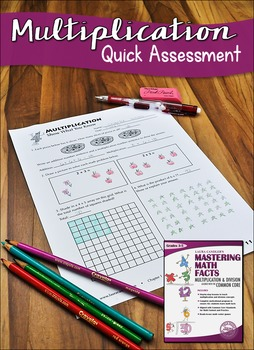# Mastering Multiplication Facts: Lessons, Activities, Games, and Assessments3rd - 5th, Homeschool
Subjects
Standards
Resource Type
Formats Included
• PDF
• EBooks
Pages
208 pages

#### Also included in

1. Mastering Math Facts Multiplication and Division, Graphic Organizers for Reading, and Power Reading Workshop are included in one cost-saving bundle. All three ebooks by Laura Candler feature easy-to-follow lessons, ready-to-use printables, and management strategies designed to ensure your success.
\$29.95
\$44.85
Save \$14.90
2. Mastering Math Facts Multiplication and Division: Aligned with the Common Core and Power Reading Workshop: A Step-by-step Guide are paired in this bundle. Both digital books by Laura Candler include complete, easy-to-follow lessons, ready-to-use printables and management strategies to ensure your su
\$22.95
\$29.90
Save \$6.95
3. Mastering Math Facts Multiplication and Division: Aligned with the Common Core and Graphic Organizers for Reading: Teaching Tools Aligned with the Common Core are paired together in this Common Core Reading and Math Bundle. Both books by Laura Candler include step-by-step lessons that make it easy t
\$22.95
\$29.90
Save \$6.95

### Description

Frustrated because your students don't know their times tables? Looking for specific teaching strategies for teaching and reinforcing multiplication and division facts? Mastering Math Facts is a comprehensive resource that includes dozens of lessons, strategies, games, and activities to introduce or review multiplication and division facts. The step-by-step lessons make it easy to teach the foundational concepts of multiplication and division, and the Mastering Math Facts motivational system ensures that all students learn their math facts fluently. If you follow this program as written, your students WILL learn the times tables fluently! Click the preview link above to see every page in this ebook!

Save with a bundle purchase!
Mastering Math Facts can also be purchased at a discount as a part of one of the bundles below.
• Power Reading Workshop and Mastering Math Facts Ebook Bundle
• Graphic Organizers for Reading and Mastering Math Facts Ebook Bundle
• Laura Candler Reading and Math Ebook Bundle
Total Pages
208 pages
Included
Teaching Duration
3 months
Report this Resource to TpT
Reported resources will be reviewed by our team. Report this resource to let us know if this resource violates TpT’s content guidelines.

### Standards

to see state-specific standards (only available in the US).
Fluently multiply and divide within 100, using strategies such as the relationship between multiplication and division (e.g., knowing that 8 × 5 = 40, one knows 40 ÷ 5 = 8) or properties of operations. By the end of Grade 3, know from memory all products of two one-digit numbers.
Understand division as an unknown-factor problem. For example, find 32 ÷ 8 by finding the number that makes 32 when multiplied by 8.
Apply properties of operations as strategies to multiply and divide. Examples: If 6 × 4 = 24 is known, then 4 × 6 = 24 is also known. (Commutative property of multiplication.) 3 × 5 × 2 can be found by 3 × 5 = 15, then 15 × 2 = 30, or by 5 × 2 = 10, then 3 × 10 = 30. (Associative property of multiplication.) Knowing that 8 × 5 = 40 and 8 × 2 = 16, one can find 8 × 7 as 8 × (5 + 2) = (8 × 5) + (8 × 2) = 40 + 16 = 56. (Distributive property.)
Determine the unknown whole number in a multiplication or division equation relating three whole numbers. For example, determine the unknown number that makes the equation true in each of the equations 8 × ? = 48, 5 = __ ÷ 3, 6 × 6 = ?.
Use multiplication and division within 100 to solve word problems in situations involving equal groups, arrays, and measurement quantities, e.g., by using drawings and equations with a symbol for the unknown number to represent the problem.